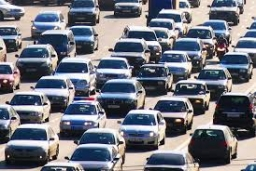# Calculate 9571

At 9:00 AM, a car left Zlín in the direction of Brno, 98 km away. At 9:15 AM, a vehicle left Brno in the direction of Zlín and drove at a speed 6 km/h higher than the speed of the car traveling from Zlín. The cars met at 9:45 AM. Calculate the speeds of both cars.

z =  76 km/h
b =  82 km/h

### Step-by-step explanation:

z·(9+45/60-9.00)+b·(9+45/60-(9+15/60))=98
b = z+6

30b+45z = 5880
b-z = 6

Row 2 - 1/30 · Row 1 → Row 2
30b+45z = 5880
-2.5z = -190

z = -190/-2.5 = 76
b = 5880-45z/30 = 5880-45 · 76/30 = 82

b = 82
z = 76

Our linear equations calculator calculates it.Did you find an error or inaccuracy? Feel free to write us. Thank you!

Tips for related online calculators
Do you have a linear equation or system of equations and looking for its solution? Or do you have a quadratic equation?
Do you want to convert length units?
Do you want to convert velocity (speed) units?
Do you want to convert time units like minutes to seconds?